Win up to 100% scholarship on Aakash BYJU'S JEE/NEET courses with ABNAT Win up to 100% scholarship on Aakash BYJU'S JEE/NEET courses with ABNAT

# Image Formation in Concave Mirror

In this lesson, we will discuss image formation in concave mirrors. However, before we delve into the topic, let us quickly recall what a concave mirror is and the principles behind its working. A concave mirror is a type of spherical mirror in which the reflecting surface is the inner-curved surface of the sphere, i.e., in this type of mirror, the reflecting surface seems to be away from the incident light source. Because of their shape, the incident light is reflected inwards (converged); thus, they are also called converging mirrors and are used for focusing light.

Both laws of reflection are valid at every point on the curved surface of the mirror. The normal is drawn along the radius, i.e., it is drawn by joining the centre of curvature of the mirror to the point of incidence. The convergence of the rays after reflection is because the normal to the reflecting surface differs at each point on the mirror.

There is a formation of different images in a concave mirror. It mainly depends on the distance between the object and the mirror. Concave mirrors form both real and virtual images. When the concave mirror is placed very close to the object, a virtual and magnified image is obtained, and if we increase the distance between the object and the mirror, the size of the image reduces and real images are formed. These real images can be projected on a screen. The focal point and the centre of curvature of the concave mirror lie in front of the mirror.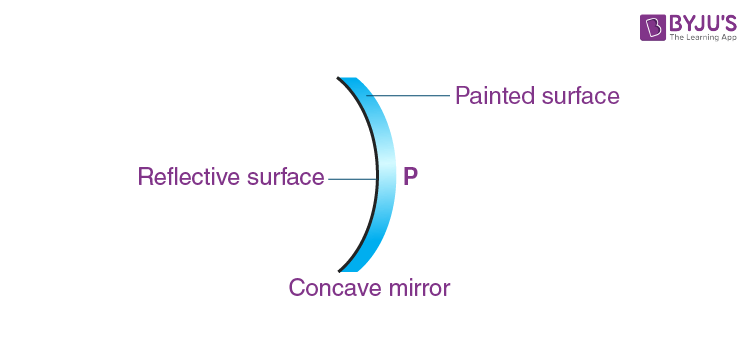## Image Formation Important Terms

1. Pole: It is the centre of the reflecting surface of a spherical mirror. It lies on the surface of the mirror, and it is usually denoted by P.
2. Centre of curvature: The centre of the sphere formed by the reflecting part of a spherical mirror is called the centre of curvature. It is generally denoted by C. This is not a part of the mirror, and it lies outside the reflecting surface of the mirror. In a concave mirror, it lies in front of the mirror.
3. The radius of curvature: It is the radius of the sphere formed by the reflecting part of the sphere. It is represented by R.
4. Principal axis: It is the straight line passing through the pole and centre of curvature of the spherical mirror. This is normal to the mirror at its pole.
5. Principal focus: The incident rays coming parallel to the principal axis after reflection appear to converge to a common point on the principal axis, and this point is called the principal focus of a concave mirror. It is usually denoted by F.
6. Focal length: It is the distance between the pole and the principal focus of the concave mirror. It is denoted by f.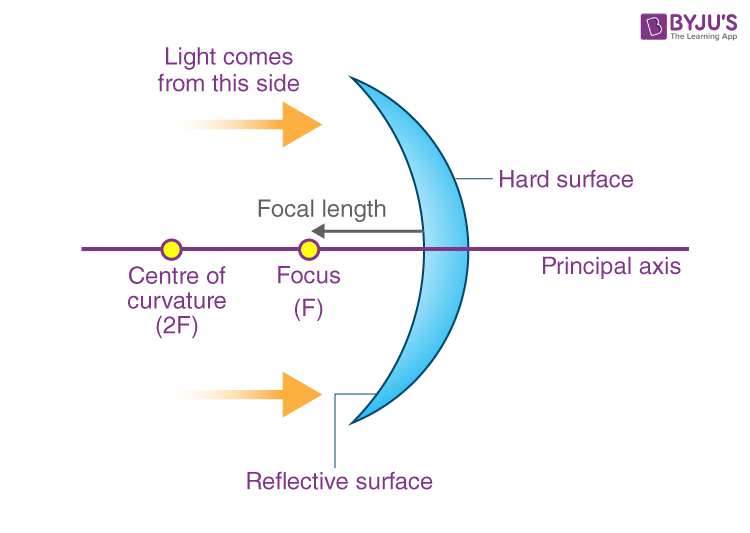## Concave Mirror Ray Diagrams

Ray diagrams are necessary for understanding the formation of an image by a concave mirror. For constructing ray diagrams and for a better understanding of image formation, we should consider at least two incident rays coming from the object. The intersection of these two rays after reflection gives the position of the image of the object. For a concave mirror, any of the following four ray diagrams can be used for locating the image formed:

a) A ray parallel to the principal axis, after reflection, will pass through the principal focus of a concave mirror.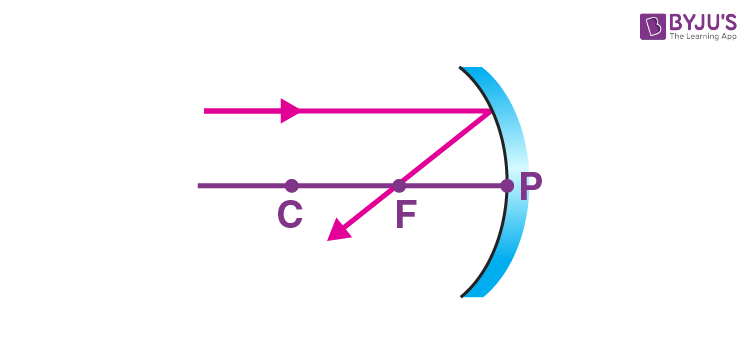b) A ray which is passing through the principal focus of a concave mirror, after reflection, will emerge parallel to the principal axis.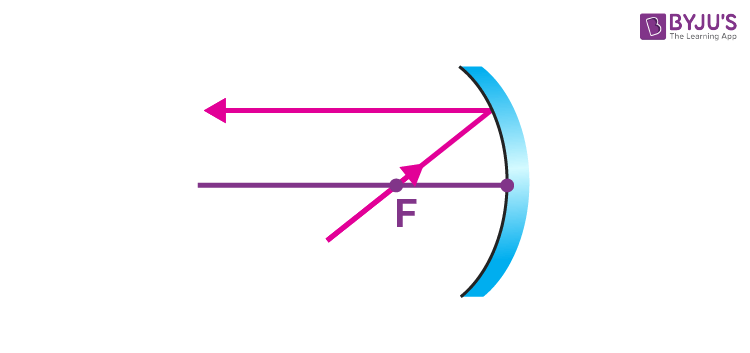c) A ray passing through the centre of curvature of a concave mirror, after reflection, is reflected along the same path. The light rays come back along the same path because the incident rays fall on the mirror along the normal to the reflecting surface.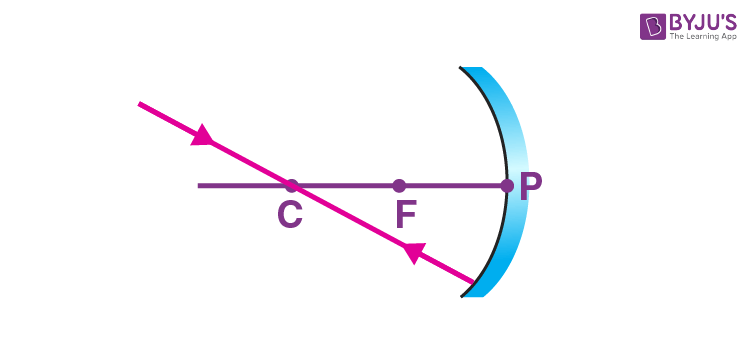d) A ray incident obliquely to the principal axis, towards the point P (pole of the mirror), on the concave mirror, is reflected obliquely. The incident and reflected rays follow the laws of reflection at point P, making equal angles with the principal axis.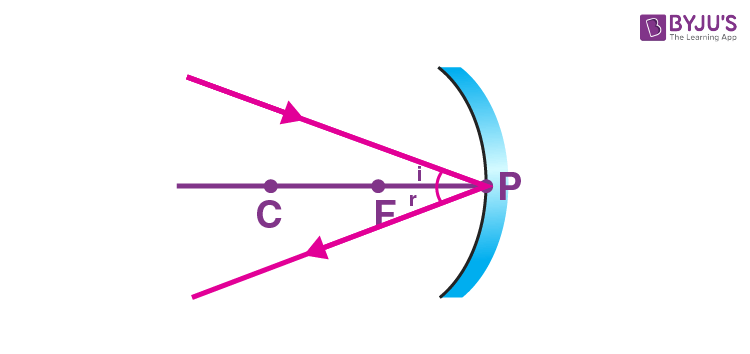## Concave Mirror Image Formation

Rays emerging from a point meet at another point after reflection, and this point is called the image of the first point. The image is real if the rays converge to the point, and it is virtual if the rays do not meet it, but appear to diverge from a point when the rays are produced backwards. During image formation, we assume that the rays are paraxial, i.e., they are incident at points close to the pole P of the mirror and make small angles with the principal axis. For a concave mirror, we consider six positions of the object before the mirror.

1. When the object is placed at infinity

2. When the object is placed beyond C (centre of curvature)

3. When the object is placed at C

4. When the object is placed between C and F (principal focus)

5. When the object is placed at F

6. When the object is placed between F and P (pole)

### When the Object Is at Infinity

In this condition, we consider two rays parallel to the principal axis originating from the object. These rays, after reflection, converge and form an image at F, the principal focus of the mirror, in front of the mirror. The image thus formed is highly diminished, point size, real and inverted.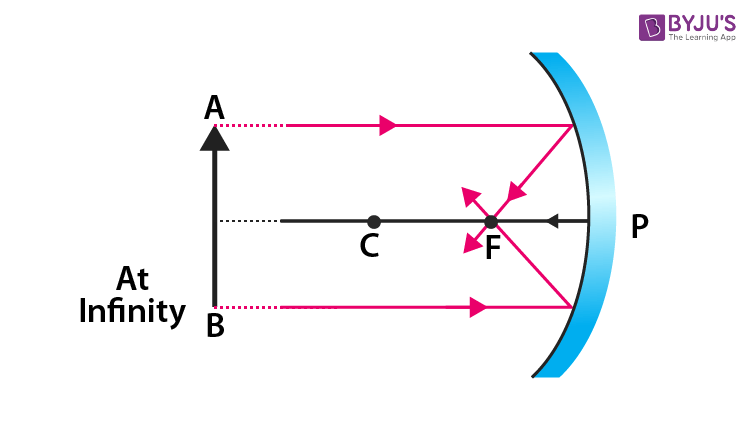### The Object Is Placed Beyond C

In this situation, we consider two different rays emerging from the object. One is parallel to the principal axis, and the other is directed towards the centre of curvature of the mirror. These rays, after reflection, form an image between the centre of curvature (C) and the focus (F). The image thus formed is diminished, real and inverted.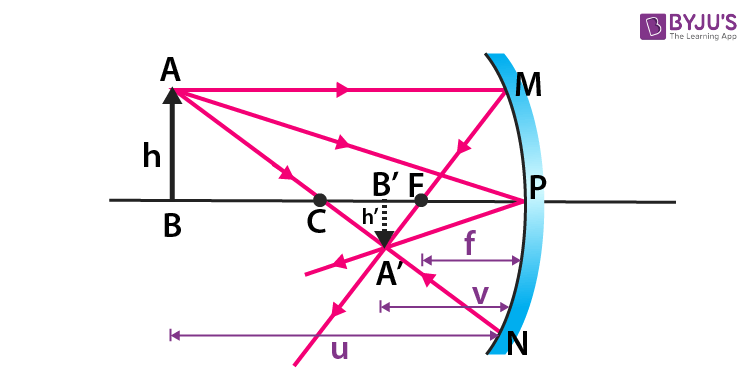### The Object Is Placed at C

Here, the two rays emerging from the object are one parallel to the principal axis and the other passing through the focus of the mirror. These rays, after reflection, form an image at point C. The image formed has the same size as that of the object, and it is real and inverted.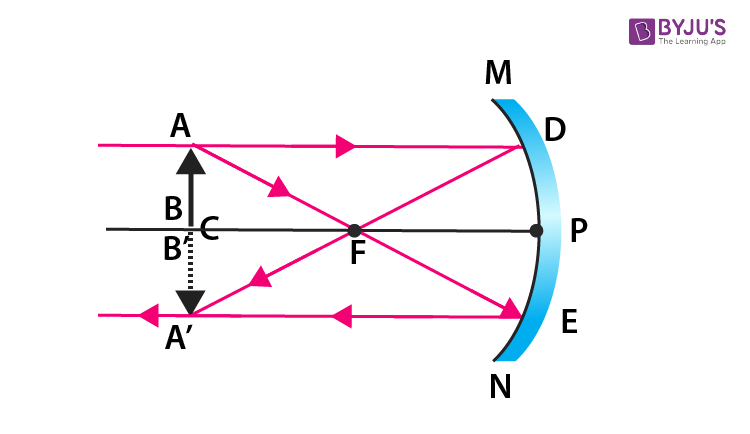### The Object Is Placed between C and F

Here, the two rays considered are one parallel to the principal axis and the other passing through the principal focus of the concave mirror. The image is formed beyond C. The image is larger compared to the size of the object, and it is real and inverted.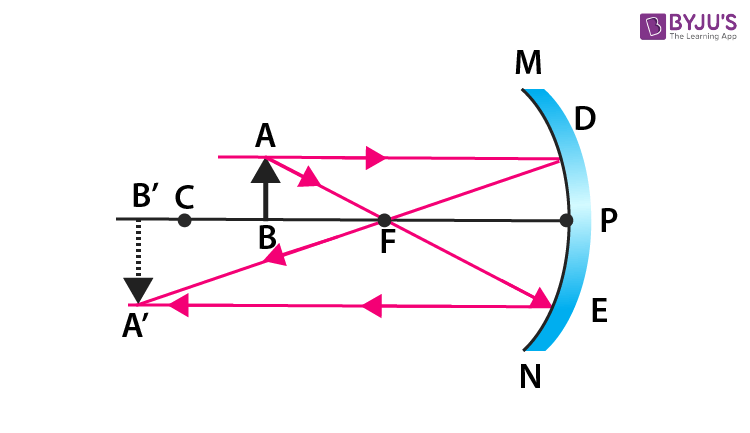### The Object Is Placed at F

The rays considered here are one parallel to the principal axis and the other passing through the centre of curvature of the mirror. This results in the formation of a highly enlarged image which is real and inverted at infinity.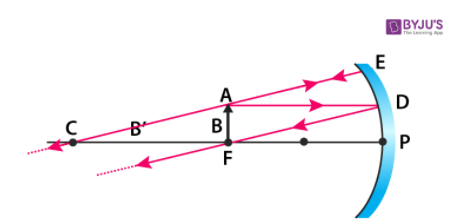### The Object Is Placed between F and P

The rays considered here are one going parallel to the principal axis and the other passing through the centre of curvature of the mirror. The image formed here is virtual and erect, and it is larger than the object.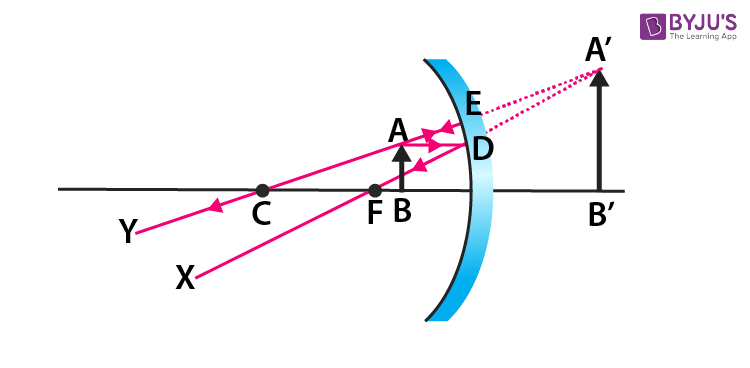## Image Formation Tabular Data

 Position of the Object Position of the Image Size of the Image Nature of the Image At infinity At focus, F Highly diminished and pointed in size Inverted and real Beyond C Between F and C Diminished Inverted and real At C At C Same size Inverted and real Between C and F Beyond C Enlarged Inverted and real At F At infinity Highly enlarged Inverted and real Between F and P Behind the mirror Enlarged Erect and virtual

Also Read: Image Formation in Convex Mirror

## Concave Mirror Sign Conventions

For deriving the relevant formulas for reflection by spherical mirrors, there is a standard sign convention for measuring distances. The normally used convention is the Cartesian sign convention. According to this convention, all the distances are measured from the pole of the mirror, i.e., the pole (P) of the mirror is assumed as the origin. The principal axis of the mirror is taken as the x-axis of the coordinate system. The conventions are as given below:

• The object is always placed on the left side of the mirror. This means that the light from the object falls on the mirror from the left-hand side.
• All the distances which are parallel to the principal axis are measured from the pole of the mirror.
• All the distances measured towards the right of the origin (along + x-axis) are taken as positive and while those measured to the left of the origin (along – x-axis) are taken as negative, or the distances measured in the same direction as that of the incident light are taken as positive and those measured in the direction opposite to the direction of incident light are taken as negative.
• Distances measured perpendicular to and above the principal axis of the mirrors are taken as positive.
• Distances measured perpendicular to and below the principal axis of the mirrors are taken as negative.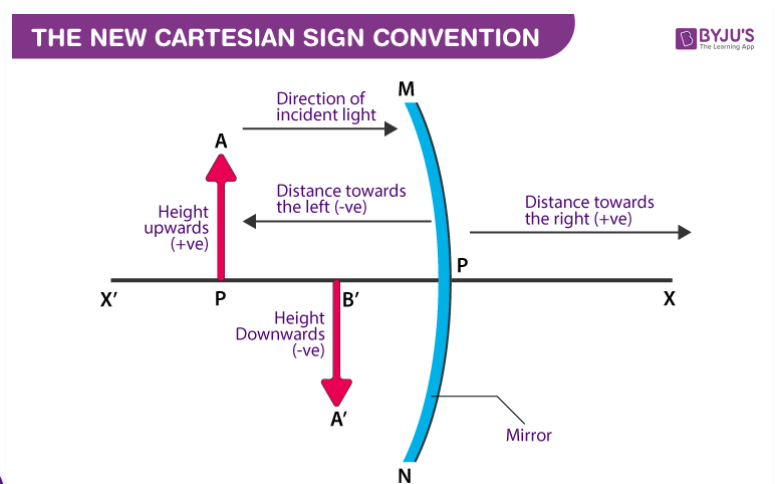## Relation between Radius of Curvature and Focal Length

Consider a ray parallel to the principal axis striking the concave mirror at a point M on its reflecting surface. Then CM will be perpendicular to the mirror at point M. Let θ be the angle of incidence, and MD be the perpendicular from M to the principal axis.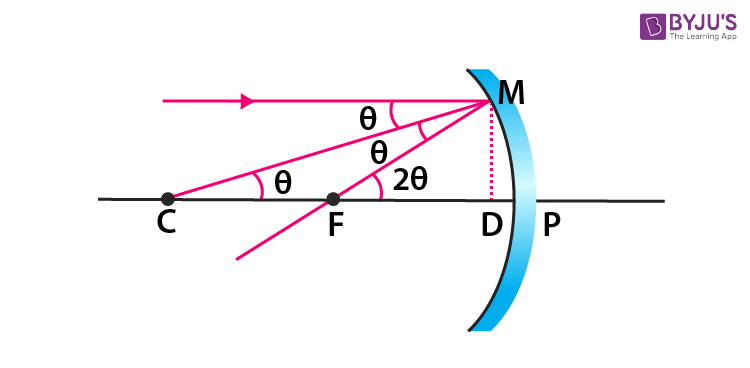Then from the figure,

∠MCP = θ and ∠MFP = 2θ

Now,

Tanθ = MD / CD and tan2θ = MD / FD

For small values of θ, which is true for paraxial rays, tan θ ≈ θ and tan 2θ ≈ 2θ

Therefore, MD / FD = 2 X MD / CD or FD = CD / 2

Now, FD = f and CD = R, therefore the above equations become

f = R / 2

This is from the assumption that for small values of θ point, D is very close to point P.

## Mirror EquationThe figure shows two rays emerging from the object. These rays, after reflection, form an image A’B’. From the geometry of ray diagrams, the two right-angled triangles ABF and MPF are similar. This is because, for paraxial rays, the line MP can be considered to be a straight line perpendicular to CP. Therefore,

BA / PM = BF / FP or BA / BA = BF / FP (Since PM = AB) ………………… (1)

Since ∠APB = ∠A’PB’, the right-angled triangles A’B’P and ABP are also similar,

BA / BA = BP / BP ……………… (2)

Comparing the equations (1) and (2), we get,

BF / FP = BP – FP / FP = BP / BP

This is the relationship involving the magnitude of distances.

In a spherical mirror, the distance of the object from its pole is called the object distance (u) and the distance of the image from the pole is called the image distance (v). As mentioned earlier, the distance of the principal focus from the pole is called the focal length (f).

Substituting u, v, and f by following the sign convention, we get,

-v + f / -f = -v / -u or v – f / f = v / u or 1 / u + 1 / v = 1 / f

This equation is known as the mirror equation. This is valid for both convex and concave mirrors.

## Linear Magnification (m)

Linear magnification (m) is the ratio of the height of the image (h’) to the height of the object (h).

m = h / h

h’ and h are given a positive or negative value as per the Cartesian sign convention.

In triangles A’B’P and ABP, we have

BA / BA = BP / BP

By applying sign convention, we get

m = h / h = – v / u

A negative sign for the value of magnification indicates that the image is real, and a positive sign indicates that the image is virtual.

## Concave Mirror Solved Questions

1. An object is found to be 5 cm in front of a concave mirror with a radius of curvature of 15 cm. Determine the position, nature, and magnification of the image in each case.

focal length, f = =15/2 cm = 7.5 cm

u = -5 cm

Applying the mirror formula, we get,

1 / V + I / -5 = 1 / -7.5

1 / V = I / -7.5 + 1 / 5 = 1 / 15

Therefore, v =15 cm

Thus, a virtual and erect image is formed 15 cm behind the mirror.

Magnification, m = – v / u = 15 / -5 = 3

Thus, the image formed is virtual, erect and magnified by a factor of 3.

2. An object, 4 cm in size, is placed at 25 cm in front of a concave mirror of focal length 15 cm. At what distance from the mirror should a screen be placed to obtain a sharp image? Determine the nature and the size of the image.

Object size, h = + 4 cm

Object distance, u = – 25 cm

Focal length, f = –15 cm

By using the mirror equation,

1 / V = I / -15 – 1 / -25 = – 1 / 15 + 1 / 25

1 / V = -5 + 3 / 75 = 2 / 75

i.e. v = – 38.5 cm

To get the image on the screen, the screen should be placed at a distance of 38.5 cm from the mirror.

Magnification, m = h / h = – v / u

Therefore,

h = – vh / u = (-38.5 x 4) / -25 = 6.16

Height of the image, h’ = 6 cm, so the image formed is real, inverted and enlarged.

## Uses of Concave Mirrors

Concave mirrors are used in many instances. Some of the common ones are given below.

• Torches, searchlights and vehicle headlights use concave mirrors to get powerful parallel beams of light.
• Shaving mirrors used are usually concave mirrors to get a magnified image of the face.
• To see large images of the teeth of patients, dentists use concave mirrors.
• Concave mirrors are also used in reflecting telescopes.
• Concave mirrors are used to form optical cavities, which are important in the construction of lasers.
• For concentrating sunlight to produce heat in solar furnaces, large concave mirrors are used.
• Concave mirrors are used as the mirror landing aid system of modern aircraft carriers.

#### Video Lesson – Paraxial Rays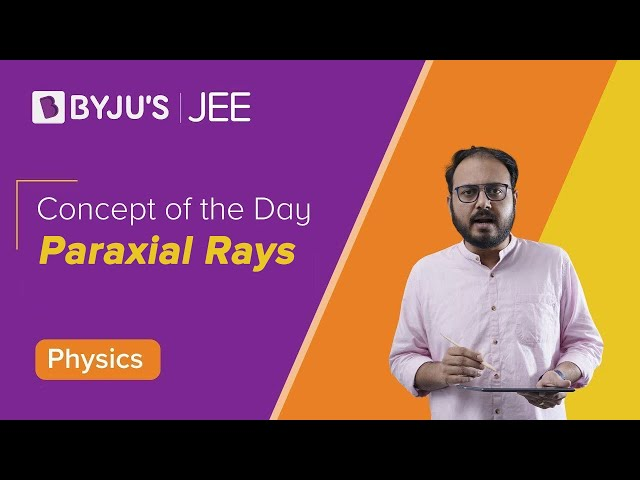#### Ray Optics – Total Internal Reflection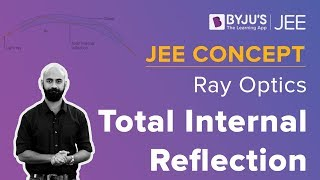Ray Optics – Important JEE Questions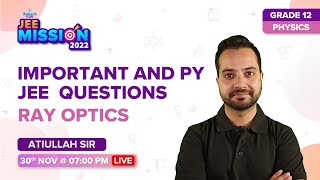#### Ray Optics – Most Important Topics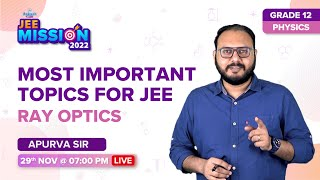#### Ray Optics – Prism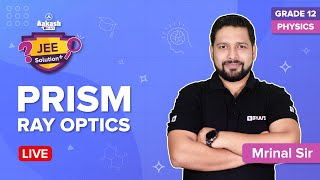#### Ray Optics – Refraction through Lens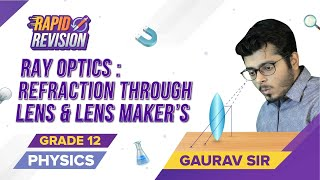#### Ray Optics – Lens Combination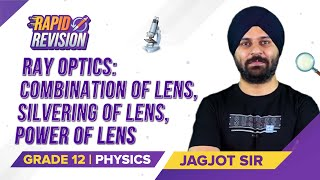#### Ray Optics – Refraction through Spherical Surface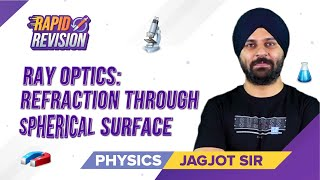Lens Maker’s Formula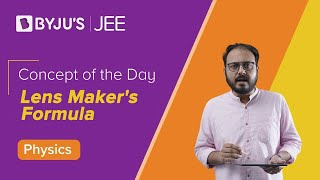## Frequently Asked Questions on Image Formation in Concave Mirror

Q1

### What are the uses of concave mirrors in daily life?

Concave mirrors are used in torches, telescopes, head mirrors, solar furnaces, headlights, etc.

Q2

### What types of images are formed by a concave mirror?

Both real and virtual images are formed by a concave mirror.

Q3

### Is the focal length of the concave mirror positive or negative?

The focal length of the concave mirror is negative.

Q4

### What is a concave mirror?

The concave mirror has a reflecting surface bent inwards.

Test Your Knowledge on Concave Mirror Image Formation